# How To Find The Perimeter Of A Triangle (3 Things To Know)

Finding the perimeter of a triangle can help you to figure out how much material you need to build a structure. You can also compare the distance you would need to walk around two different triangles to see which is greater.

So, how do you find the perimeter of a triangle?  To find the perimeter of a triangle, take the sum of the three side lengths. If one or two side lengths are missing (unknown), use the Law Of Cosines, Law Of Sines, Pythagorean Theorem, Special Triangles, or the coordinates of the vertices to help you find the missing side lengths.

Of course, the methods you can use to find missing side lengths will depend on the type of triangle (e.g. right triangle or not) and also the information you are given.

In this article, we’ll talk about how to find the perimeter of a triangle when missing various pieces of information.  We’ll also look at several examples to make the concepts clear.

Let’s get started.

## How To Find The Perimeter Of A Triangle

To find the perimeter of a triangle, we add up the three side lengths. The formula is:

• P = a + b + c

where P is the perimeter of the triangle and a, b, c, are the side lengths of the triangle.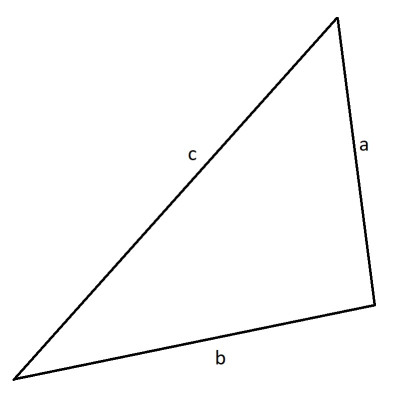Here we see a triangle with side lengths a, b, c. The perimeter P = a + b + c.

Here is an example.

### Example: How To Find The Perimeter Of A Triangle

Let’s say we have the triangle pictured below.

The side lengths are a = 2, b = 3, and c = 4. The perimeter formula tells us that:

• P = a + b + c
• P = 2 + 3 + 4
• P = 9

So, the perimeter of the triangle is 9 units.

The formula for perimeter of a triangle is straightforward to apply when we have all three side lengths, as in the example above.

However, we often need to find one or more side lengths with information we are given. Let’s look at some of those scenarios now.

### How To Find The Perimeter Of A Triangle With Coordinates

If we are given the coordinates of the three vertices (“corners”) of a triangle, we can still find the area. We just need to use the vertices to find the three side lengths, and then apply the same perimeter formula as above.

Remember that the distance D between two points (x1, y1) and (x2, y2) is given by the distance formula:

• D = √((x2 – x1)2 + (y2 – y1)2)

(This formula comes from the Pythagorean Theorem).

The three side lengths of the triangle pictured above would be given by the formulas:

• a = √((x2 – x1)2 + (y2 – y1)2)
• b = √((x3 – x1)2 + (y3 – y1)2)
• c = √((x3 – x2)2 + (y3 – y2)2)

Here is an example.

#### Example: How To Find The Perimeter Of A Triangle With Coordinates

Let’s say you have a triangle with the following coordinates: (3, 2), (3, 5), and (7, 2), as shown below.

We will assign:

• (x1, y1) = (3, 2)
• (x2, y2) = (3, 5)
• (x3, y3) = (7, 2)

Using the formula above for the side length of a, we get:

• a = √((x2 – x1)2 + (y2 – y1)2)
• a = √((3 – 3)2 + (5 – 2)2)
• a = √((0)2 + (3)2)
• a = √(0 + 9)
• a = √9
• a = 3

Similarly, for b we get:

• b = √((x3 – x1)2 + (y3 – y1)2)
• b = √((7 – 3)2 + (2 – 2)2)
• b = √((4)2 + (0)2)
• b = √(16 + 0)
• b = √(16)
• b = 4

Finally, for c, we get:

• c = √((x3 – x2)2 + (y3 – y2)2)
• c = √((7 – 3)2 + (2 – 5)2)
• c = √((4)2 + (-3)2)
• c = √(16 + 9)
• c = √(25)
• c = 5

Now that we have the three side lengths a = 3, b = 4, and c = 5, we can find the perimeter with the formula from before:

• P = a + b + c
• P = 3 + 4 + 5
• P = 12

### How To Find The Perimeter Of A Triangle With One Side Missing

If we have two sides of the triangle, but one side is missing, we can still find the perimeter if we know the angle across from the missing side.

The method in this case is to use the Law Of Cosines.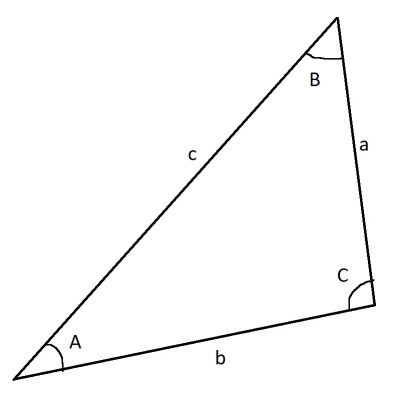This triangle has side lengths a, b, and c, with corresponding angle measures A, B, and C.

For the triangle with side lengths and angles in the image above, the Law Of Cosines says that:

• a2 + b2 – 2abcos(C) = c2

Of course, we can also switch up the sides and angles used in the formula to get two other formulas:

• a2 + c2 – 2accos(B) = b2
• b2 + c2 – 2bccos(A) = a2

If one side is missing and we have an angle other than the one across from the missing side, then use the Law Of Sines to find the angle across from the missing side, then use the Law Of Cosines.

Let’s try some examples.

#### Example 1: How To Find The Perimeter Of A Triangle With One Side Missing (Law Of Cosines)

Let’s say that we have the triangle pictured below, with two known side lengths and the angle between them (included angle) known: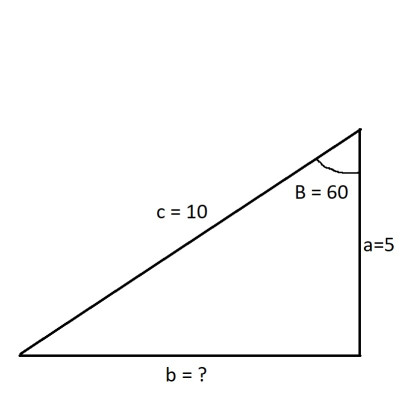This triangle has side lengths a = 5, c = 10, and b unknown, with an angle of B = 60 degrees across from the unknown side length b.

Then we have a = 5, c = 10, B = 60 degrees, and b is unknown.

Using the 2nd formulation for the Law Of Cosines above, we get:

• a2 + c2 – 2accos(B) = b2
• 52 + 102 – 2(5)(10)cos(60) = b2
• 25 + 100 – 100(0.5) = b2
• 125 – 50 = b2
• 75 = b2
• √75 = b
• 5√3 = b

Now that we have b, we can find the perimeter:

• P = a + b + c
• P = 5 + 5√3 + 10
• P = 15 + 5√3
• P = 5(3 + √3)

Now we’ll try a similar example, but with a twist: we will need to use the Law Of Sines as well.

Remember that the Law of Sines says that:

• sin(A)/a = sin(B)/b = sin(C)/c

So, if we have a pair of sides and the corresponding pair of angles, we only need three of the four and we can find the fourth with the Law Of Sines.

#### Example 2: How To Find The Perimeter Of A Triangle With One Side Missing (Law Of Sines & Law Of Cosines)

Let’s say that we have the triangle pictured below, with two known side lengths and the angle across from the smallest side known: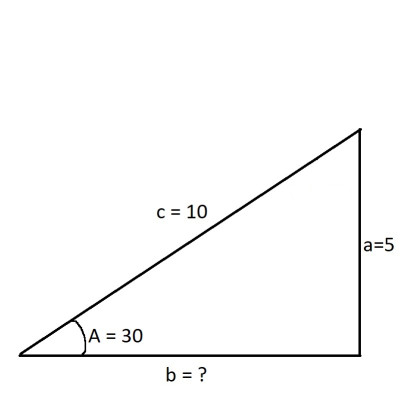This triangle has side lengths a = 5, c = 10, and b unknown, with an angle of A = 30 degrees across from the known side length a.

Then we have a = 5, c = 10, A = 30 degrees.

Using the Law Of Sines, we have:

• sin(A)/a = sin(C)/c
• sin(30)/5 = sin(C)/10
• 0.5/5 = sin(C)/10
• 0.5(10) = 5sin(C)  [by cross multiplication]
• 5 = 5sin(C)
• 1 = sin(C)
• 90 = C

Remember that the sum of the three angles in a triangle is 180 degrees, so:

• A + B + C = 180
• 30 + B + 90 = 180
• B + 120 = 180
• B = 60

Now that we have the angle B, we can use the same Law Of Cosines calculation from the previous example to find b = 5√3.

As above:

• P = a + b + c
• P = 5 + 5√3 + 10
• P = 15 + 5√3
• P = 5(3 + √3)

### How To Find The Perimeter Of A Triangle With Two Sides Missing

In certain cases, we can find the perimeter of a triangle with two sides missing. For example:

• For an equilateral triangle, all of the side lengths are the same. So, if one side length is given, we just multiply it by 3 to find the perimeter of the triangle.
• For a special triangle, we can use ratios of side lengths to find missing sides. For example, a 30-60-90 triangle has side lengths in the ratio 1:√3:2. So, if the shortest side has a length of 7, then the other two sides have lengths of 7√3 and 14.
• For a triangle where one side and two angles are given, we can find the third angle (sum of three angles is 180 degrees) and then use the Law Of Sines to solve for the two missing sides.

Let’s look at an example of this last case.

#### Example: How To Find The Perimeter Of A Triangle With Two Sides Missing (Law Of Sines)

Let’s say that we have the triangle pictured below, with one known side length and two angles known: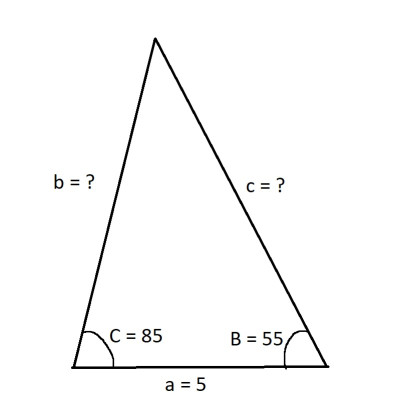This triangle has a known side length of a = 5, with known angles B = 55 degrees and C = 85 degrees. The side lengths b and c are unknown.

Then we have a = 5, B = 55 degrees, and C = 85 degrees.

Remember that the sum of the three angles in a triangle is 180 degrees, so:

• A + B + C = 180
• A + 55 + 85 = 180
• A + 140 = 180
• A = 40

Now that we have the angle A, we can use the Law Of Sines to find B and C.

Using the Law Of Sines, we can find the side length b:

• sin(A)/a = sin(B)/b
• sin(40)/5 = sin(55)/b
• bsin(40) = 5sin(55)  [by cross multiplication]
• b = 5sin(55)/sin(40)
• b = 5(0.8191)/0.6428
• b = 6.3719

Similarly, we can use the Law Of Sines to find the side length c:

• sin(A)/a = sin(C)/c
• sin(40)/5 = sin(85)/c
• csin(40) = 5sin(85)  [by cross multiplication]
• c = 5sin(85)/sin(40)
• c = 5(0.9962)/0.6428
• c = 7.7490

Now that we have b and c, we can find the perimeter of the triangle:

• P = a + b + c
• P = 5 + 6.3719 + 7.7490
• P = 19.1209

### How To Find The Third Side Of A Triangle If Perimeter Is Given

To find the third side of a triangle when perimeter is given, we just use the original formula and plug in the values we know, then solve for the one we don’t.

#### Example: How To Find The Third Side Of A Triangle If Perimeter Is Given

Let’s say we have the triangle with side lengths a = 4, b = 5, and perimeter P = 16.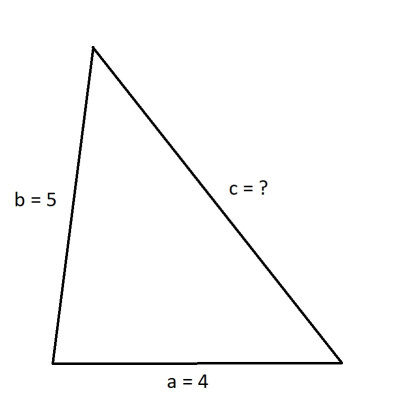This triangle has known side lengths a = 4 and b = 5, with perimeter 16. The side length of side c is unknown.

To find the third side length c, we use the perimeter formula:

• P = a + b + c
• 16 = 4 + 5 + c
• 16 = 9 + c
• 7 = c

## How To Find The Perimeter Of A Right Triangle

When finding the perimeter of a right triangle, we can use the Law Of Sines, but we can also use the Pythagorean Theorem (which is a special case of the Law Of Cosines when one of the angles is 90 degrees).

• If you know any two sides in a right triangle, you can find the third one by the Pythagorean Theorem: a2 + b2 = c2.
• If you know one side and one angle (besides the 90 degree angle) in a right triangle, then you can find the third angle and then use the Law Of Sines to find the other side lengths.

### Example 1: How To Find The Perimeter Of A Right Triangle With One Side Missing (Given Two Side Lengths)

Let’s say we have the right triangle with side lengths a = 5 and b = 12, pictured below.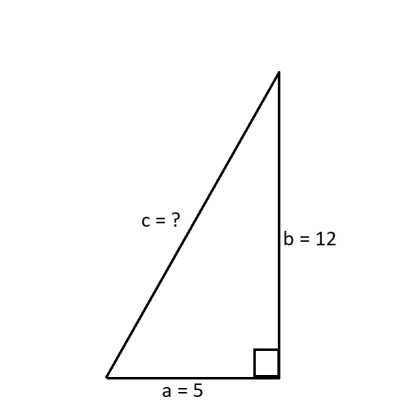This right triangle has a 90-degre angle and two known side lengths of a = 5 and b = 12. The third side length c is unknown.

By the Pythagorean Theorem, we can find the third side length c:

• a2 + b2 = c2
• 52 + 122 = c2
• 25 + 144 = c2
• 169 = c2
• 13 = c

Now, we use the perimeter formula:

• P = 5 + 12 + 13
• P = 30

### Example 2: How To Find The Perimeter Of A Right Triangle With Two Sides Missing (Given One Side Length & One Angle)

Let’s say we have the right triangle with side length a = 5 and angle B = 40, pictured below.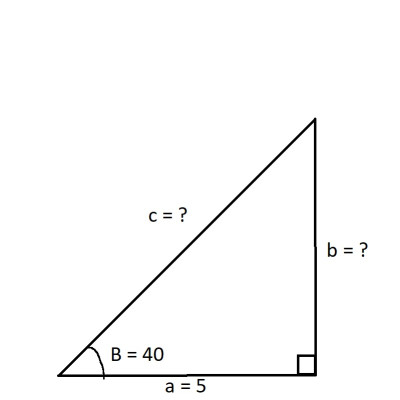This right triangle has a 90 degree angle, and angle of B = 40 degrees, and a side length of a = 5. The side lengths b and c are unknown.

Remember that the sum of the three angles in a right triangle is 180, and one of the angles is 90 degrees by definition, so:

• A + B + C = 180
• A + 40 + 90 = 180
• A + 130 = 180
• A = 50

Now that we have the angle A, we can use the Law Of Sines to find the side lengths b and c.

To find b:

• sin(A)/a = sin(B)/b
• sin(50)/5 = sin(40)/b
• bsin(50) = 5sin(40)  [by cross multiplication]
• b = 5sin(40)/sin(50)
• b = 5(0.6428)/0.7660
• b = 4.1950

To find c, we can use the Law Of Sines or the Pythagorean Theorem.  We’ll use Law Of Sines:

• sin(A)/a = sin(C)/c
• sin(50)/5 = sin(90)/c
• sin(50)/5 = 1/c  [sin(90) = 1]
• 5/sin(50) = c  [take reciprocals of both sides]
• 5/0.7660 = c
• 6.5270 = c

Now we can find the perimeter:

• P = a + b + c
• P = 5 + 4.195 + 6.5270
• P = 15.7220

## Conclusion

Now you know how to find the perimeter of a triangle, even when missing one or more side lengths to begin with.

You can learn when triangles are similar here.

You can learn about uses of triangles in real life here.